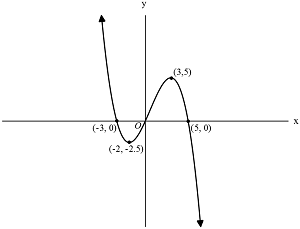# SAT FunctionsThe function $f$ is shown above. What are all the values of $x$ for which $f$ is negative?

(A) $\ \ -\infty < x< -3\ \text{and}\ 0 < x < 5$
(B) $\ \ -\infty < x < -2$
(C) $\ \ -3 < x <0\ \text{and}\ 5 < x < \infty$
(D) $\ \ -3 < x < 0$
(E) $\ \ 0 < x < 5$

×